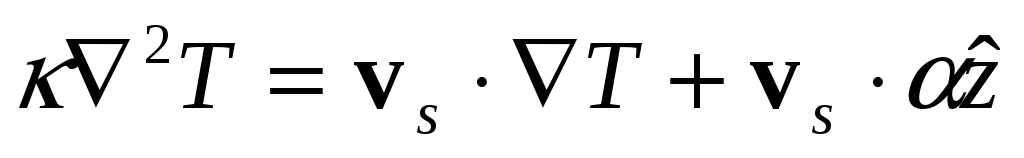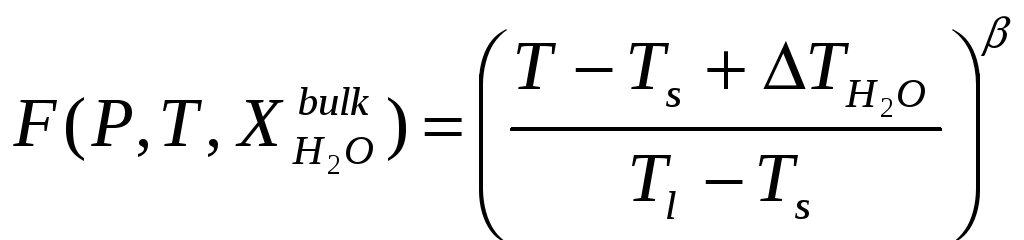# Supplementary Information

 Page 1/12 Date 26.11.2017 Size 1.58 Mb. #35345

## Supplementary Information

We carried out numerical experiments to estimate the extent to which the upper mantle is cooled by a long-offset, low-slip transform, such as the Romanche. The temperature field before the onset of melting has been calculated by the steady-state advection-diffusion equation: 

where =mantle thermal diffusivity, 8.04 10-7 m2/s; vs=matrix velocity vector; =adiabatic temperature gradient, 0.0003 °C/m and z=unit vector along z-axis. Mantle temperatures have been computed through a 3D-domain of mantle flow calculations, by the over-relaxation upwind finite difference method described by ref. 1, using a variable grid spacing (512x256x101) with the highest grid resolution (1 km) in the proximity of the plate boundaries. Temperature solutions were found assuming constant temperature at the surface (0 °C) and different mantle potential temperatures at 150 km depth in order to evaluate the Equatorial MAR cold spot effect. Melt parameters of the model (crustal thickness and maximum degree of melting) were inferred from the chemistry of basalts sampled from the centre of the ERRS2. A 1330 °C mantle potential temperature has been assumed at a depth of 150 km beneath the ERRS, 50°C colder than "normal" (temperature that produces 6 km of crust, assuming that all melt is extracted).

Mantle flow velocities were estimated in equation (1) assuming steady-state plate thickening passive flow3,4. We modeled the corner flow induced by seafloor spreading in a computational frame 2048x1024 km wide and 150 km deep (2x2 km spaced grid points for each 1 km depth increment) assuming an incompressible, homogeneous, isoviscous mantle beneath a Romanche-like ridge-transform-ridge plate boundary geometry (offset 900 km) with a spreading rate of 16 mm/yr. We solved for the steady-state three-dimensional passive mantle flow via a Fourier pseudo-spectral technique. The base of rigid plates, assumed to correspond to the depth of 700 °C isotherm, was obtained iteratively solving each time the mantle temperature field, starting from a constant-thickness plate-flow model. A 512 km long ridge segment, longer than the ERRS, was chosen in order to evaluate how far the transform effect extends along axis, avoiding numerical edge effects

Water in the upper mantle plays an important role in governing melt generation beneath spreading centres5. The amount of water present in the oceanic upper mantle is sufficient to deepen the peridotite solidus6 and cause partial melting in a region wider and deeper than that expected for an anhydrous mantle7,8. We modeled melt generation, including the effect of water on the peridotite solidus, using a modified form of a recent parameterization of experimental data developed by ref. 9, adding a pressure-dependent water bulk distribution coefficient and near-fractional melting regimes. The total amount of melting (F) can be expressed as a function of pressure (P), temperature (T) and weight fraction of water dissolved in the melt:(2)

where Ts and Tl are the temperatures of the solidus and the lherzolite liquidus, respectively;